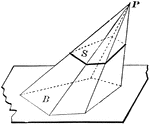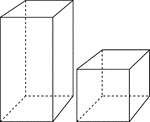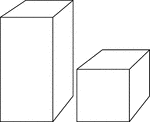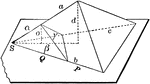### Pyramid Cut By Plane

Diagram used to prove the theorem: "If a pyramid is cut by a plane parallel to the base, the edges are…### 2 Rectangular Prisms

Illustration of 2 right rectangular prisms. The bases are congruent, but the height of the smaller prism…### 2 Rectangular Prisms

Illustration of 2 right rectangular prisms. The bases are congruent, but the height of the smaller prism…### Two Proportional Tetrahedrons

Diagram used to prove the theorem: "Two tetrahedrons having a trihedral angle in each equal, are to…